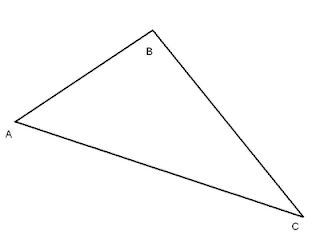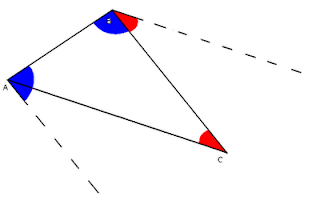## Thursday, November 11, 2010

### Thinking Aloud

I noticed on a recent quiz that my kids have trouble identifying angle relationships once there is a network of more than 3 lines. I have an idea for making kids construct parallel lines using the angle concepts, that will hopefully help to further their ability to visualize angle relationships.

In my mind, the exercise looks like this:

1. I'll first let the kids draw a scalene triangle and label it ABC.2. I'll ask the kids to use a protractor to construct "a line parallel to AC at point B, using the concept of alternate interior angles." (The kids should be able to do this quickly, since that's the same angle relationship we used during our tessellations project a while back to create parallel lines. But, in my experience, kids need some help interpreting things like "a line parallel to AC at B." It's surprisingly difficult for them to decode what that means!)3. Then, I'll ask the kids to construct "a line parallel to BC at point A, using the concept of same-side interior angles."4. Finally, the kids will construct "a line parallel to AB at point C, using the concept of corresponding angles."5. Depending on if the kids feel like this has been a difficult exercise or not, at this point I might optionally insert requirements for written explanations next to each newly constructed line, explaining which angle pairs are which type of angles. (Good practice with naming angles with 3 letters.)

Just thinking aloud.

#### 1 comment:

1.There's lots of good stuff you can do with this. Example. If we call the point of intersection of the first two parallels D, then ABDC is a parallelogram. Its diagonal divides it into congruent triangles whose area must be the same. But if we know already that a pgram has area of base times height, we see that, for any triangle, its area is half base times height.

Another example. One pair alt int: ABC and DCB. A second pair: DBC and ACB. Angle addition gives us that angles ABD and ACD are congruent. Third angle theorem proves that angle BAC = angle BDC. Conclusion: in a pgram, opp angles are congruent.

A final example. Extend side AB upwards. Another pair of alt int angles are created. The three angles at B sum to 180. Thus the three angles of triangle BCD sum to 180. Bingo - the triangle angle sum theorem.

Fun fun.